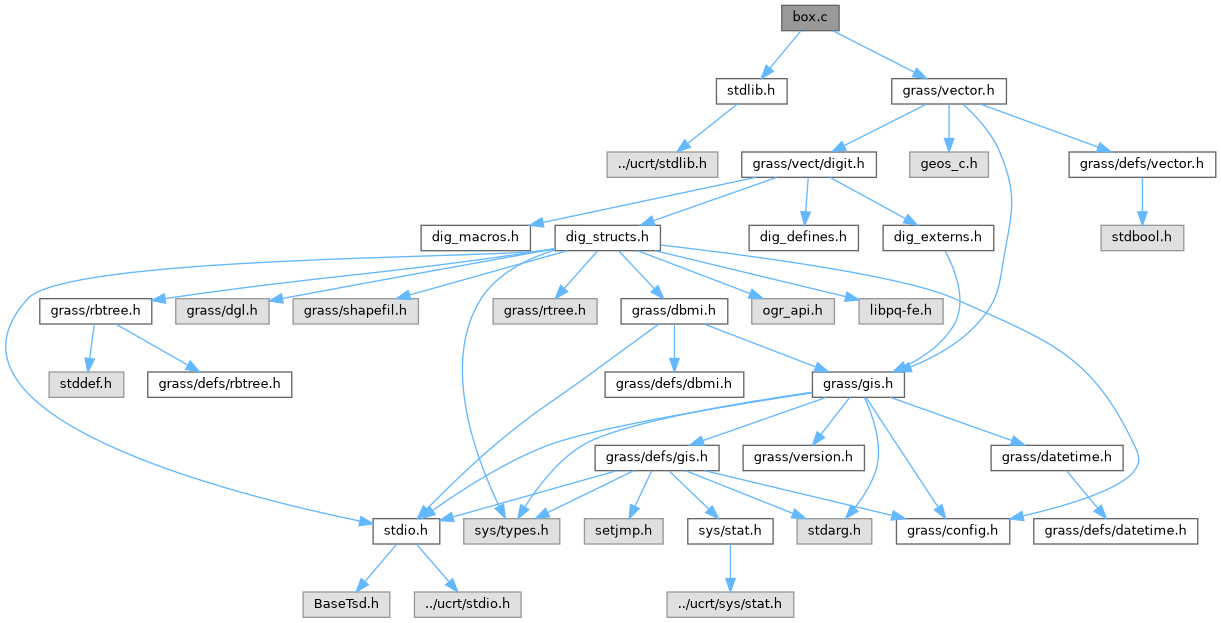GRASS GIS 8 Programmer's Manual  8.2.2dev(2023)-5c00b4e944
vector/diglib/box.c File Reference
`#include <stdlib.h>`
`#include <grass/vector.h>`
Include dependency graph for vector/diglib/box.c:Go to the source code of this file.

## Functions

int dig_line_box (const struct line_pnts *Points, struct bound_box *Box)

int dig_box_copy (struct bound_box *A, struct bound_box *B)

int dig_box_extend (struct bound_box *A, struct bound_box *B)

## ◆ dig_box_copy()

 int dig_box_copy ( struct bound_box * A, struct bound_box * B )

Definition at line 64 of file vector/diglib/box.c.

## ◆ dig_box_extend()

 int dig_box_extend ( struct bound_box * A, struct bound_box * B )

Definition at line 81 of file vector/diglib/box.c.

## ◆ dig_line_box()

 int dig_line_box ( const struct line_pnts * Points, struct bound_box * Box )

Definition at line 24 of file vector/diglib/box.c.

Referenced by Vect_build_line_area().